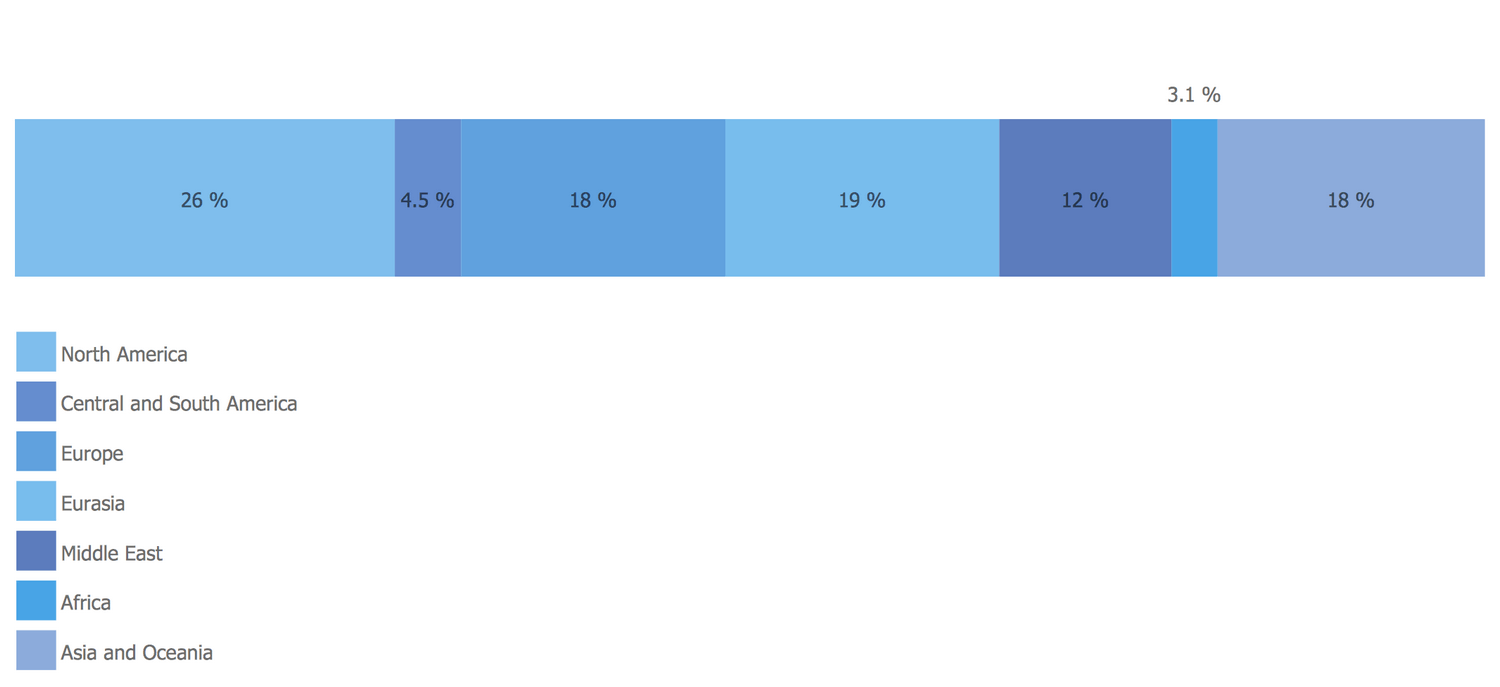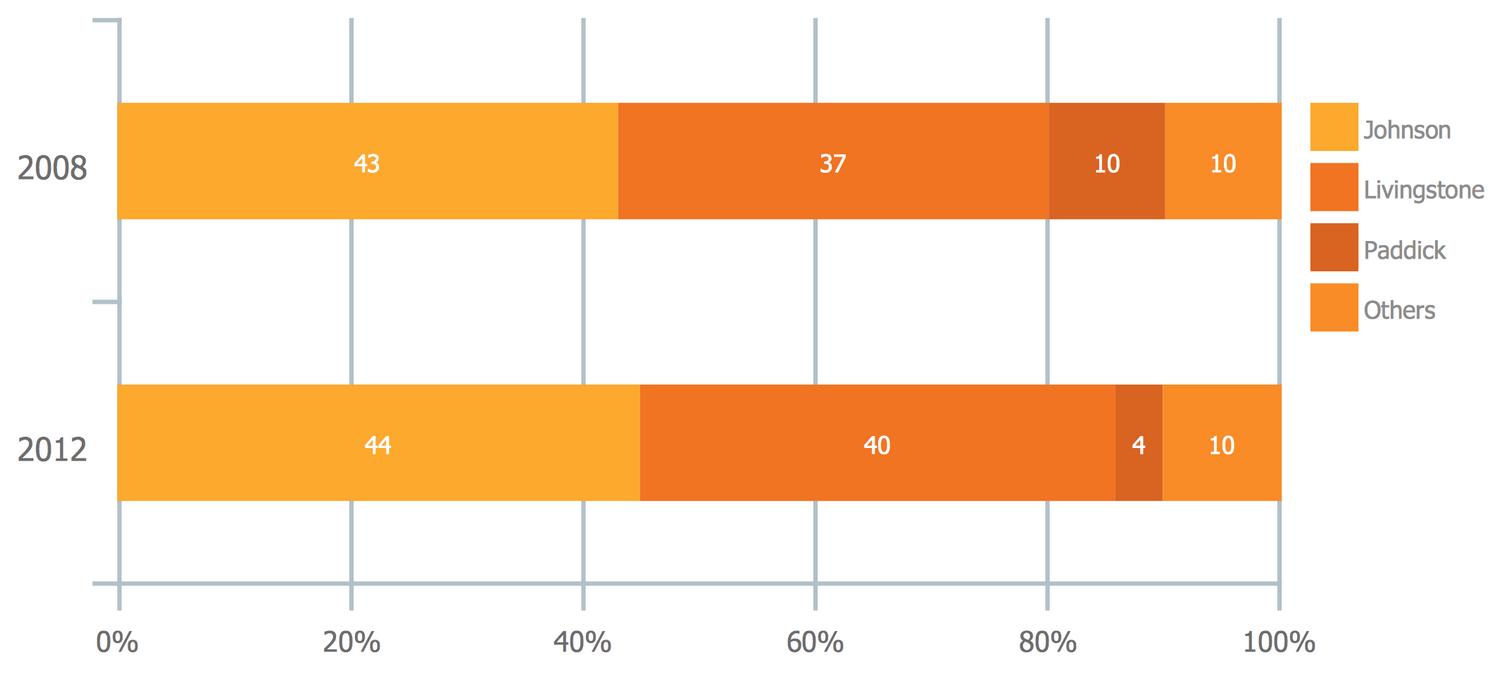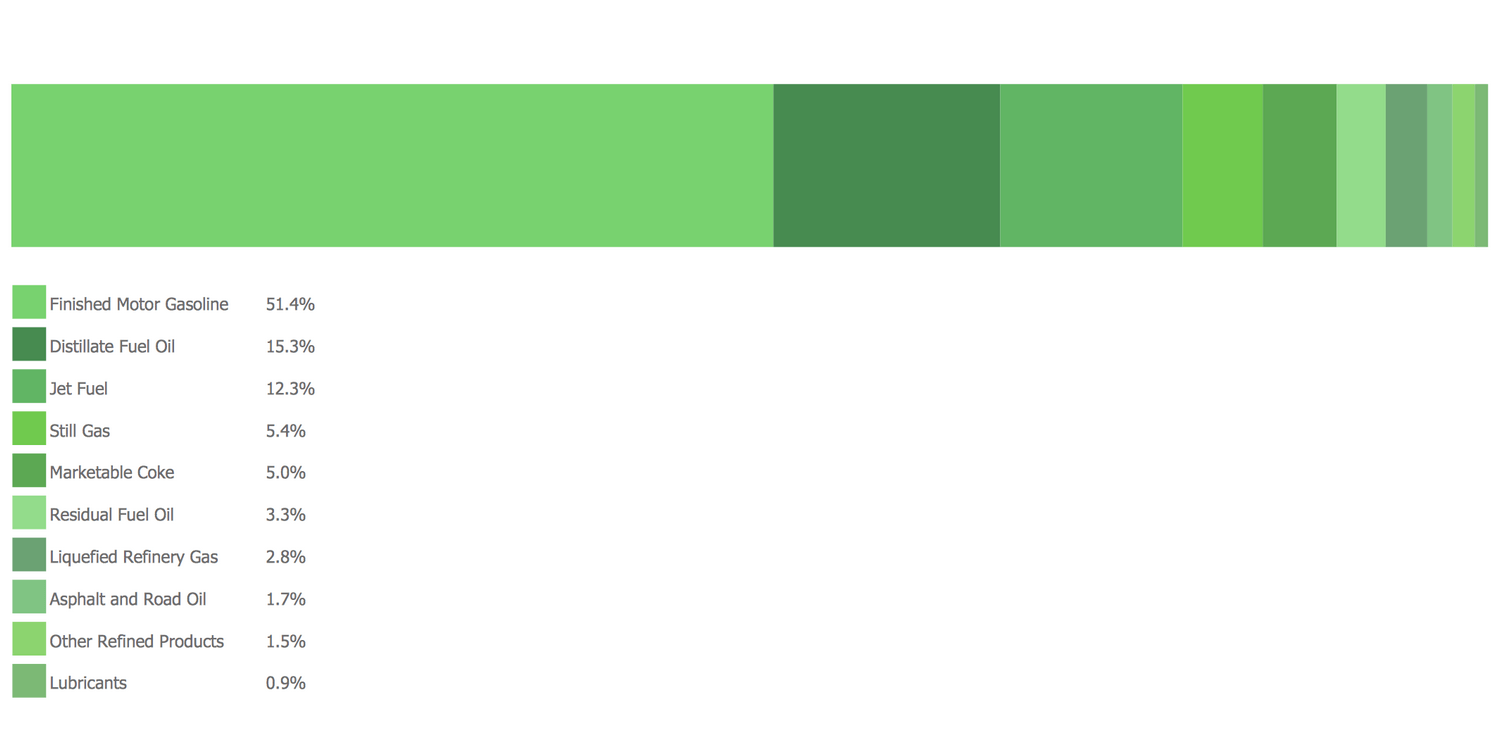This site uses cookies. By continuing to browse the ConceptDraw site you are agreeing to our Use of Site Cookies.
ConceptDraw Samples | Graphs and Charts — Basic Divided Bar Diagrams

# Basic Divided Bar Diagrams

Samples of bar and column charts are created using ConceptDraw DIAGRAM diagramming and vector drawing software and the Basic Divided Bar Diagrams solution.

ConceptDraw DIAGRAM provides export of vector graphic multipage documents into multiple file formats: vector graphics (SVG, EMF, EPS), bitmap graphics (PNG, JPEG, GIF, BMP, TIFF), web documents (HTML, PDF), PowerPoint presentations (PPT), Adobe Flash (SWF).

## Tutorials and Solutions:

Video Tutorials: ConceptDraw Solution Park

Solutions: Basic Divided Bar Diagrams for ConceptDraw DIAGRAM

## Sample 1: Basic Divided Bar Diagram Composition of Estates

Basic Divided Bar Diagrams sample: Composition of Estates.

This example is created using ConceptDraw DIAGRAM diagramming software enhanced with Basic Divided Bar Diagrams from ConceptDraw Solution Park.## Sample 2: Global Natural Gas Consumption

Basic Divided Bar Diagrams sample: Global Natural Gas Consumption.

This example is created using ConceptDraw DIAGRAM diagramming software enhanced with Basic Divided Bar Diagrams from ConceptDraw Solution Park.## Sample 3: Basic Divided Bar Diagram — London Election Results

Basic Divided Bar Diagrams sample: London Election Results.

This example is created using ConceptDraw DIAGRAM diagramming software enhanced with Basic Divided Bar Diagrams from ConceptDraw Solution Park.## Sample 4: Products Yielded from One Barrel of Crude Oil

Basic Divided Bar Diagrams sample: Products Yielded from One Barrel of Crude Oil.

This example is created using ConceptDraw DIAGRAM diagramming software enhanced with Basic Divided Bar Diagrams from ConceptDraw Solution Park.All samples are copyrighted CS Odessa's.

Usage of them is covered by Creative Commons “Attribution Non-Commercial No Derivatives” License.

The text you can find at: https://creativecommons.org/licenses/by-nc-nd/3.0